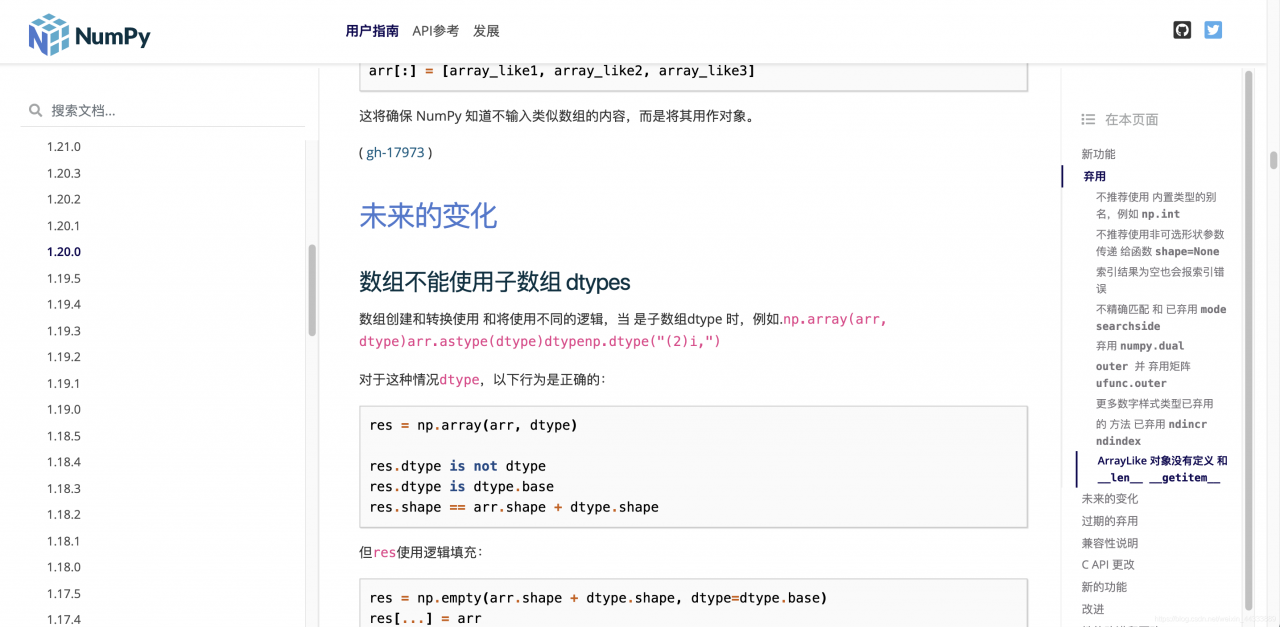# For the problem of rejecting old usage errors after numpy is updated, modified in numpy 1.20; for more details and guidance

There’s something wrong with numpy

Because the latest version of numpy is updated today, the previous usage is invalid

So You need to use the latest usage

Originally, my return value is like this

``````return np.array(df).astype(np.float)
``````

Error:

DeprecationWarning: `np.float` is a deprecated alias for the builtin
`float`. To silence this warning, use `float` by itself. Doing this
will not modify any behavior and is safe. If you specifically wanted
the numpy scalar type, use `np.float64` here.

Deprecated in NumPy 1.20; for more details and guidance: https://numpy.org/devdocs/release/1.20.0-notes.html#deprecations
return np.array(df).astype(np.float)

It seems to be because of the version of the problemIf you look at the picture
Modify to the following can be.

``````return np.array(df, dtype=float)
``````

perhaps

``````return np.array(df).astype(np.float64)
``````

So you won’t make a mistake